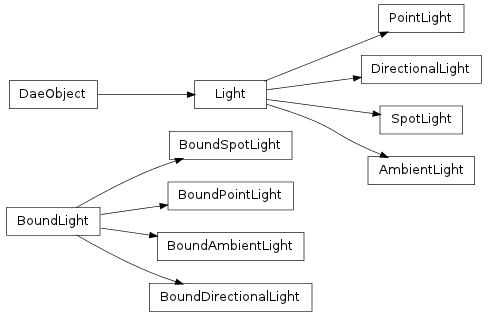#### Next topic

Point light as defined in COLLADA tag <point>.__init__(id, color, constant_att=None, linear_att=None, quad_att=None, zfar=None, xmlnode=None)

Create a new sun light.

Parameters: id (str) – A unique string identifier for the light color (tuple) – Either a tuple of size 3 containing the RGB color value of the light or a tuple of size 4 containing the RGBA color value of the light constant_att (float) – Constant attenuation factor linear_att (float) – Linear attenuation factor quad_att (float) – Quadratic attenuation factor zfar (float) – Distance to the far clipping plane xmlnode – If loaded from xml, the xml node

Methods

 __init__(id, color[, constant_att, ...]) Create a new sun light. bind(matrix) Binds this light to a transform matrix. load(collada, localscope, node) save() Saves the light’s properties back to xmlnode
id

The unique string identifier for the light

color

Either a tuple of size 3 containing the RGB color value of the light or a tuple of size 4 containing the RGBA color value of the light

constant_att

Constant attenuation factor.

linear_att

Linear attenuation factor.

zfar

Distance to the far clipping plane

xmlnode

ElementTree representation of the light.

save()

Saves the light’s properties back to xmlnode

bind(matrix)

Binds this light to a transform matrix.

Parameters: matrix (numpy.array) – A 4x4 numpy float matrix collada.light.BoundPointLight